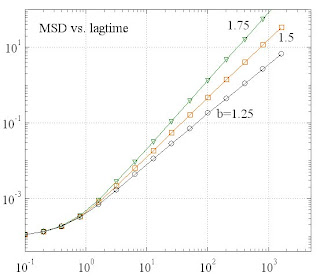## Tuesday, April 29, 2008

### [AD 14] Test of MSD and PSD in s*d model

Within the sd-model, it can be shown that the VAC depends only on the mean value d_mean and on the variance d_var=sigD2 of the step width distribution, not on the detailed distribution function. The PL-exponent is not at all affected by the step widths.

As a test, the correlation function Css(m) of the sign factors was chosen as

This form assures a smooth transition to the required value of Css(0)=sigS2=1/4. The PL regime starts at m>>1. Then, the VAC of the correlated part of the random process yields

For simplicity, it was assumed for the evaluation that

Using the discrete MSD formula from [MT 6], one finds the expected behaviour:A fast Fourier transform of Cvv yields the corresponding PSD (the frequencies are in Hz):The simulation parameters were:

dT=0.1; // simulation time intervall
NMX=16*1024; // nr. of sim. time steps

sigN2=0.5e-4; // variance of white noise
dMean=0.5e-2; // mean step width
sigD2=dMean*dMean; // variance of step width
beta=1.25, 1.5, 1.75; // PL exponent of MSD

Note that a mean step width of about 5 nm seems to be a reasonable value, in order to get the sub- to superdiffusive transition time correct.